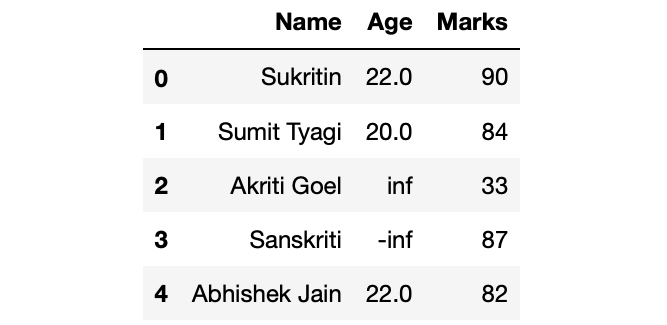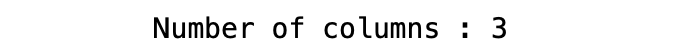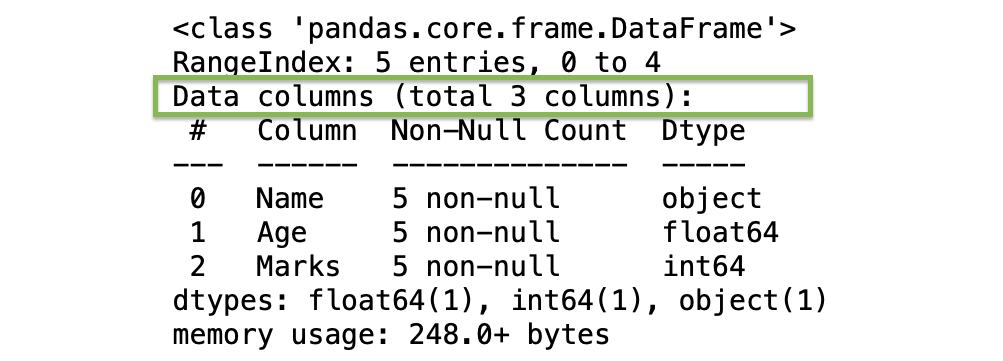Related Articles
Count number of columns of a Pandas DataFrame
• Last Updated : 26 Jul, 2020

Let’s discuss how to count the number of columns of a Pandas DataFrame. Lets first make a dataframe.

Example:

## Python3

 `# Import Required Libraries``import` `pandas as pd``import` `numpy as np`` ` `# Create a dictionary for the dataframe``dict` `=` `{``'Name'``: [``'Sukritin'``, ``'Sumit Tyagi'``, ``'Akriti Goel'``,``                 ``'Sanskriti'``, ``'Abhishek Jain'``], ``        ``'Age'``: [``22``, ``20``, np.inf, ``-``np.inf, ``22``], ``        ``'Marks'``: [``90``, ``84``, ``33``, ``87``, ``82``]}`` ` `# Converting Dictionary to Pandas Dataframe``df ``=` `pd.DataFrame(``dict``)`` ` `# Print Dataframe``df`

Output:Method 1: Using shape property

Shape property returns the tuple representing the shape of the DataFrame. The first index consists of the number of rows and the second index consist of the number of columns.

## Python3

 `# Getting shape of the df``shape ``=` `df.shape`` ` `# Printing Number of columns``print``(``'Number of columns :'``, shape[``1``])`

Output:Method 2: Using columns property

The columns property of the Pandas DataFrame return the list of columns and calculating the length of the list of columns, we can get the number of columns in the df.

## Python3

 `# Getting the list of columns``col ``=` `df.columns`` ` `# Printing Number of columns``print``(``'Number of columns :'``, ``len``(col))`

Output:Method 3: Casting DataFrame to list

Like the columns property, typecasting DataFrame to the list returns the list of the name of the columns.

## Python3

 `# Typecasting df to list``df_list ``=` `list``(df)`` ` `# Printing Number of columns``print``(``'Number of columns :'``, ``len``(df_list))`

Output:Method 4: Using info() method of DataFrame

This methods prints a concise summary of the DataFrame. info() method prints information about the DataFrame including dtypes of columns and index, memory usage, number of columns, etc.

## Python3

 `# Printing info of df``df.info()`

Output:Attention geek! Strengthen your foundations with the Python Programming Foundation Course and learn the basics.

To begin with, your interview preparations Enhance your Data Structures concepts with the Python DS Course. And to begin with your Machine Learning Journey, join the Machine Learning – Basic Level Course

My Personal Notes arrow_drop_up